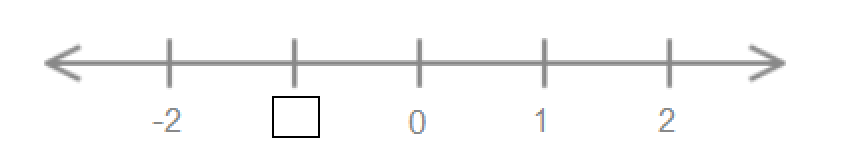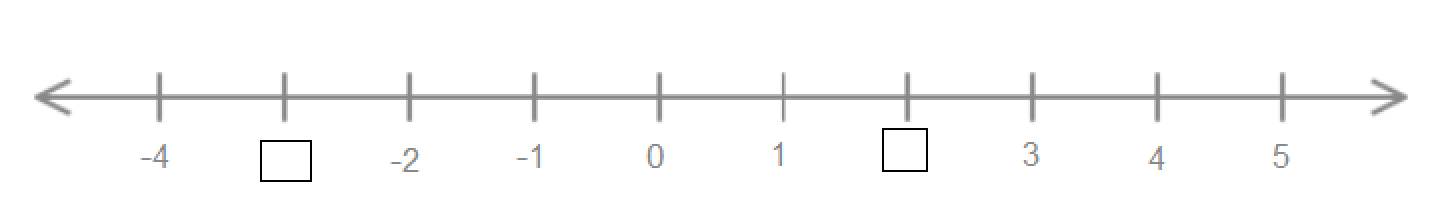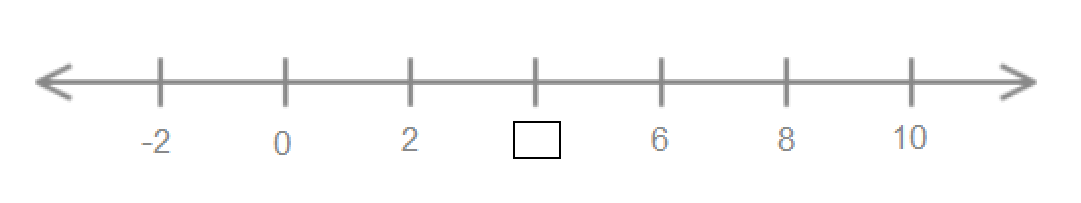# Understanding The Various Operations Of Integers

In this quiz, different questions related to different operations of integers are asked to clear the basic concept of the integers to any student. This quiz includes various multiple-choice questions to let students understand the concept of integers easily.

Start Quiz

Type the missing integer.##-1##

-1

Type the missing integers.##-3##, ##2##

2 -3

Type the missing integer.##4##

4

-4 + 3 + -5 + 6 = ##0##

0

6 + -3 + 2 + -1 = ##4##

4

5 + -12 + 16 = ##9##

9

Subtract the given integers.

2 - -3 = ##5##

5

Subtract the given integers.

-3 - -5 = ##2##

2

Is -12 x 11 positive or negative?

positive

negative

Is -2 x -5 positive or negative?

positive

negative

Quiz/Test Summary
Title: Understanding The Various Operations Of Integers
Questions: 10
Contributed by: